## Tamilnadu Samacheer Kalvi 11th Maths Solutions Chapter 12 Introduction to Probability Theory Ex 12.5

Choose the correct or most suitable answer from the given four alternatives:
Question 1.
Four persons are selected at random from a group of 3 men, 2 women and 4 children. The probability that exactly two of them are children is
(a) $$\frac{3}{4}$$
(b) $$\frac{10}{23}$$
(c) $$\frac{1}{2}$$
(d) $$\frac{10}{21}$$
Solution:
(d)
Hint:
Total no of person = 3 + 2 + 4 = 9
Selecting 4 from 9 can be done in 9 C4 ways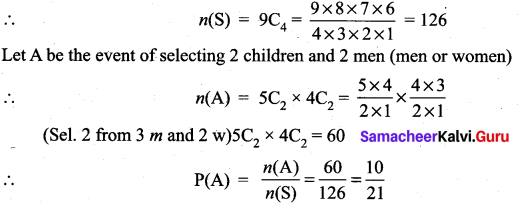Question 2.
A number is selected from the set {1, 2, 3, ….., 20}. The probability that the selected number is divisible by 3 or 4 is ……………
(a) $$\frac{2}{5}$$
(b) $$\frac{1}{8}$$
(c) $$\frac{1}{2}$$
(d) $$\frac{2}{3}$$
Solution:
(c)
Hint:
n(S) = 20
A = {3, 6, 9, 12, 15, 18} ⇒ n(A) = 6
B = {4, 8, 12, 16, 20} ⇒ n(B) = 5
A ∩ B = {12} ⇒ n(A ∩ B) = 1
so P(A ∪ B) = P(A) + P(B) – P(A ∩ B)
= $$\frac{6}{20}+\frac{5}{20}-\frac{1}{20}=\frac{10}{20}=\frac{1}{2}$$

Question 3.
A, B and C try to hit a target simultaneously but independently. Their respective probabilities of hitting the target are $$\frac{3}{4}, \frac{1}{2}, \frac{5}{8}$$. The probability that the target is hit by A or B but not by C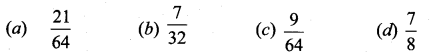Solution:
(a)
Hint:
P(A) = $$\frac{3}{4}$$ P(A’) = 1/4
P(B) = 1/2 P(B’) = 1/2
P(C) = 5/8 P(C’) = 3/8
Probability of hitting the target = 1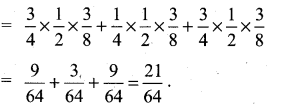Question 4.
If A and B are any two events, then the probability that exactly one of them occur is …………
(a) P(A ∪ $$\overline{B}$$) + P($$\overline{A}$$ ∪ B)
(b) P(A ∩ $$\overline{B}$$) + P($$\overline{A}$$ ∩ B)
(c) P(A) + P(B) – P(A ∩ B)
(d) P(A) + P(B) + 2P(A ∩ B)
Solution:
(b)
Hint: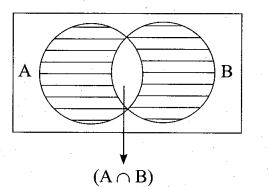Question 5.
Let A and B be two events such that P($$\overline{A \cup B}$$) = $$\frac{1}{6}$$, P(A ∩ B) = $$\frac{1}{4}$$ and P($$\overline{A}$$) = $$\frac{1}{4}$$.
Then the events A and B are …………………
(a) Equally likely but not independent
(b) Independent but not equally likely
(c) Independent and equally likely
(d) Mutually inclusive and dependent
Solution:
(b)
Hint: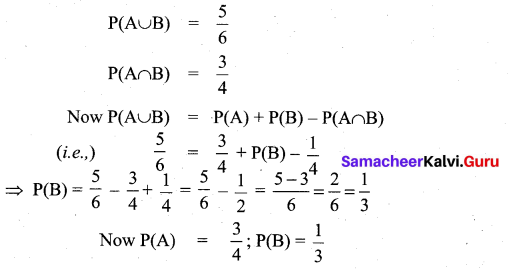So P(A). P(B) = $$\frac{3}{4} \times \frac{1}{3}=\frac{1}{4}$$
P(A ∩ B) = P(A). P(B)
⇒ A and B are independent and not equally likely

Question 6.
Two items are chosen from a lot containing twelve items of which four are defective, then the probability that at least one of the item is defective
when two items are chosen at random probability of atleast one of them is defective ………….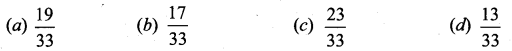Solution:
(a)
Hint:
Total number = 12
Defective = 4
∴ good ones = 12 – 4 = 8
when two items are chosen at random probability of atleast one of them is defective
= P(one defective or 2 defectives) = P(GD or DD)
= P(G) P(D) + P(D) P(D)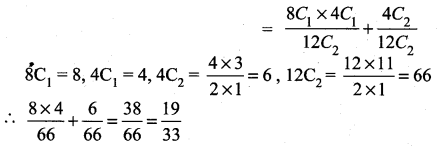Question 7.
A man has 3 fifty rupee notes, 4 hundred rupees notes and 6 five hundred rupees notes in his pocket. If 2 notes are taken at random, what are the odds in favour of both notes being of hundred rupee denomination?
(a) 1 : 12
(b) 12 : 1
(c) 13 : 1
(d) 1 : 13
Solution:
(d)
Hint: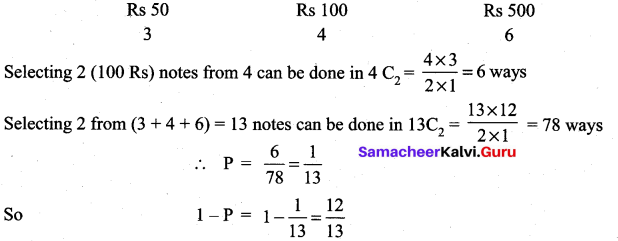The odds in favour done of P is P : 1 – P
(i.e.,) $$\frac{1}{13}: \frac{12}{13}$$ = 1 : 12

Question 8.
A letter is taken at random from the letters of the word ‘ASSISTANT’ and another letter is taken at random from the letters of the word ‘STATISTICS’. The probability that the selected letters are the same is ………….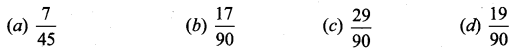Solution:
(d)
Hint: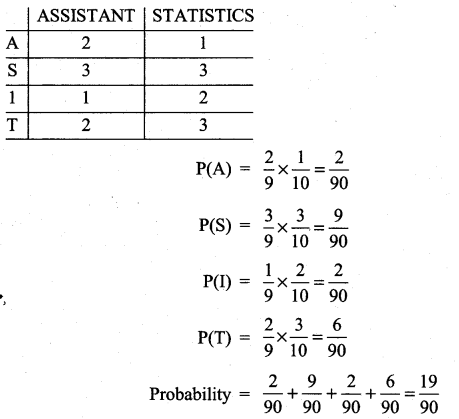Question 9.
A matrix is chosen at random from a set of all matrices of order 2, with elements 0 or 1 only. The probability that the determinant of the matrix chosen is not zero will be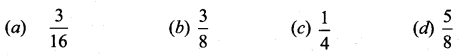Solution:
(b)
Hint: Then given elements are 0 and So each term of a matrix can be filled (Using or 1) is 2 ways.
The No. of elements is a 2 × 2 matrix = 2 × 2 = 4. So the possible ways of filling the elements of a 2 × 2 matrix is 24 = 16 (i.e.,) n(S) = 16
Let A be the event of getting a 2 × 2 matrix for which the determinant value is non zero.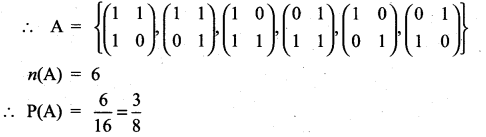Question 10.
A bag contains 5 white and 3 black balls. Five balls are drawn successively without replacement. The probability that they are alternately of different colours is …………..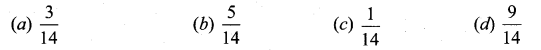Solution:
(c)
Hint: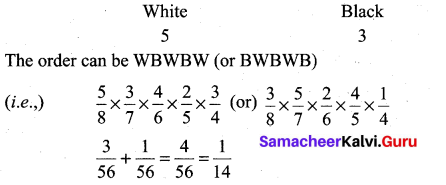Question 11.
If A and B are two events such that A ⊂ B and P(B) ≠ 0, then which of the following is correct?
(a) P(A/B) = $$\frac{\mathrm{P}(\mathrm{A})}{\mathrm{P}(\mathrm{B})}$$
(b) P(A/B) < P(A) (c) P(A/B) ≥ P(A) (d) P(A/B) > P(B)
Solution:
(c)
Hint: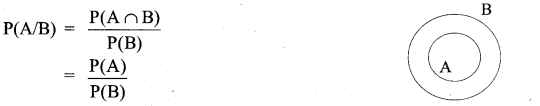Question 12.
A bag contains 6 green, 2 white, and 7 black balls. If two balls are drawn simultaneously then the probability that both are different colours is ………..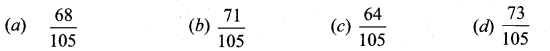Solution:
(a)
Hint: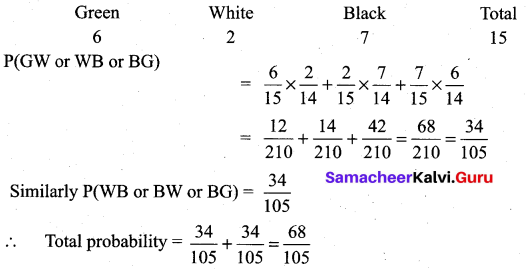Question 13.
If X and Y be two events such that P(X/Y) = $$\frac{1}{2}$$, P(Y/X) = $$\frac{1}{3}$$ and P(X ∩ Y) = $$\frac{1}{6}$$ then P(X ∪ Y) is …………
(a) $$\frac{1}{3}$$
(b) $$\frac{2}{5}$$
(c) $$\frac{1}{6}$$
(d) $$\frac{2}{3}$$
Solution:
(d)
Hint: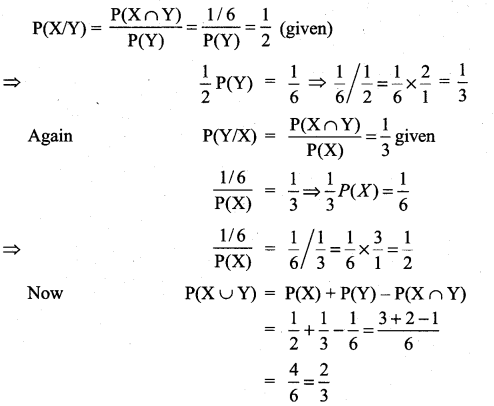Question 14.
An um contains 5 red and 5 black balls. A balls is drawn at random, its colour is noted and is returned to the um. Moreover, 2 additional balls of the colour drawn are put in the um and then a ball is drawn at random. The probability that the second ball drawn is red will be …………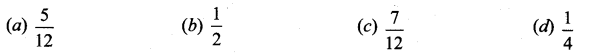Solution:
(b)
Hint: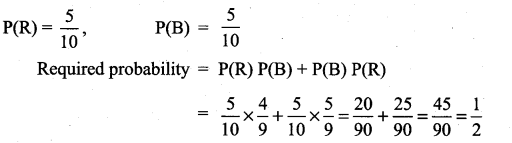Question 15.
A number x is chosen at random from the first 100 natural numbers. Let A be the event of numbers which satisfies $$\frac{(x-10)(x-50)}{x-30}$$ ≥ 0, then P(A) is …………….
(a) 0.20
(b) 0.51
(c) 0.71
(d) 0.70
Solution:
(c)
Hint: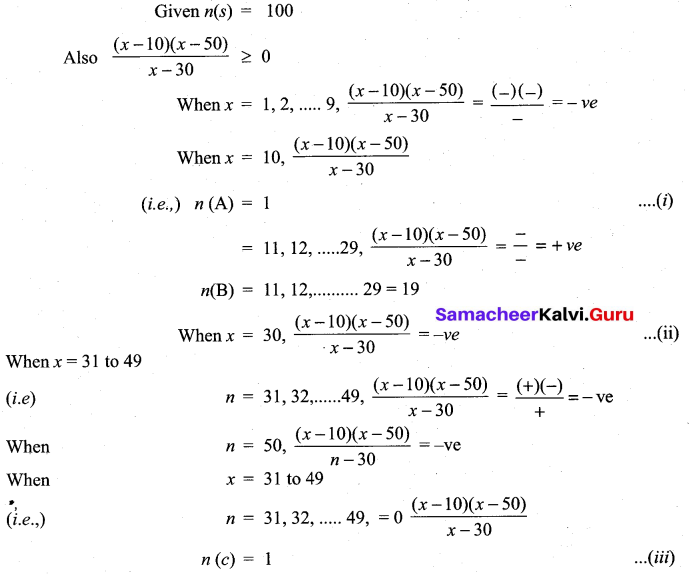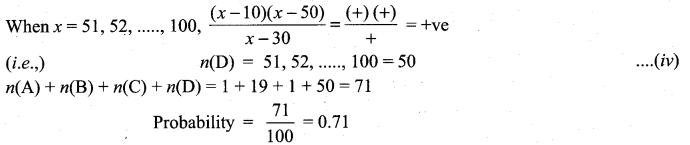Question 16.
If two events A and B are independent such that P(A) = 0.35 and P(A ∪ B) = 0.6, then P(B) is …………..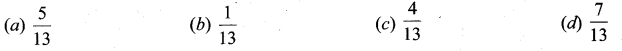Solution:
(a)
Hint:
Given A and B are independent
P(A ∩ B) = P(A) + P(B)
⇒ Now P(A ∪ B) = P(A) + P(B) – P(A ∩ B)
(i.e.,) Now P(A ∪ B) = P(A) + P(B) – P(A).P(B)
0.6 = 0.35 + P(B) – (0.35) P(B)
⇒ P(B) = (1 – 0.35) = 0.6 – 0.35
0.65 P(B) = 0.25
∴ P(B) = $$\frac{0.25}{0.65}=\frac{25}{65}=\frac{5}{13}$$Question 17.
If two event A and B are such that P($$\overline{A}$$) and P(A ∩ $$\overline{B}$$) = 1/2, then P(A ∩ B) is ………….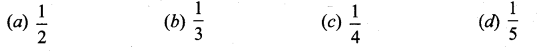Solution:
(d)
Hint: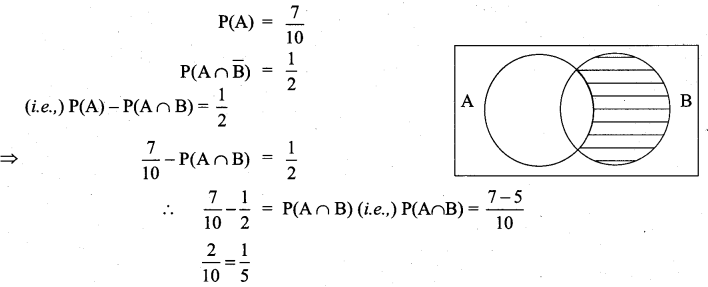Question 18.
If A and B are two events such that P(A) = 0.4, P(B) = 0.8 and P(B/A) = 0.6, then P($$\overline{A}$$ ∩ B) is …………..
(a) 0.96
(b) 0.24
(c) 0.56
(d) 0.66
Solution:
(c)
Hint:
P(B/A) = 0.6 ⇒ $$\frac{P(A \cap B)}{P(A)}$$ = 0.6
⇒ P(B/A) = 0.6 × P(A) = 0.6 × 0.4 = 0.24
Now P($$\overline{A}$$ ∩ B) = P(B) – P(A ∩ B)
= 0.8 – 0.24 = 0.56

Question 19.
There are three events A, B, and C of which one and only one can happen. If the odds are 7 to 4 against A and 5 to 3 against B, then odds against C is ……………
(a) 23 : 65
(b) 65 : 23
(c) 23 : 88
(d) 88 : 23
Solution:
(b)
Hint: If the probability of an event is P then the odds against its occurrence are 1 – P to P.
Selecting 1 from the 4 number 1, 2, 3,4, can be done in 4 ways
Here for the event A we are given that = $$\frac{1-\mathrm{P}}{\mathrm{P}}=\frac{7}{4}$$
⇒ 4 – 4P = 7P
⇒ 11 P = 4 ⇒ P = $$\frac{4}{11}$$ ⇒ P(A) = $$\frac{4}{11}$$ for the event B we are given
$$\frac{1-\mathrm{P}}{\mathrm{P}}=\frac{5}{3}$$ ⇒ 5P = 3 – 3P
⇒ 8P = 3 ⇒ P = 3/8
P(B) = $$\frac{3}{8}$$
Now we are given P(A) + P(B) + P(C) = 1
P(C) = 1 – P(A) – P(B)
P(C) = 1 – $$\frac{4}{11}-\frac{3}{8}$$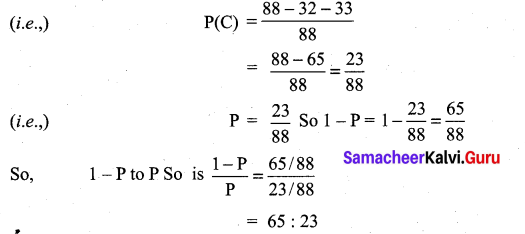Question 20.
If a and b are chosen randomly from the set {1, 2, 3, 4} with replacement, then the probability of the real roots of the equation x2 + ax + b = 0 is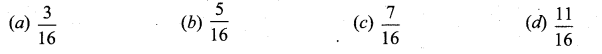Solution:
(c)
Hint:
x2 + ax + b = 0 ⇒ x = $$\frac{-a \pm \sqrt{a^{2}-4 b}}{2}$$
Given that the roots are real ⇒ a2 – 4b ≥ 0 or a2 > 4b
When a = 1, b = 1 or 2 or 3 or 4 a2 – 4b < 0
When a = 2, b = 1 a2 – 4b = 0
When a = 3, b = 1 or 2 for which a2 – 4b ≥ 0
When a = 4, b = 1 or 2, 3 or 4 for which a2 – 4b ≥ 0
So, Selecting from the 4 number 42 = 16 ways.
(i.e.,) n(s) = 16
n(A) = (2 or 3 or 4) = 3
n(B) = (1 or 2 or 3 or 4) = 4
P(A) + P(B) = $$\frac{3}{16}+\frac{4}{16}=\frac{7}{16}$$

Question 21.
It is given that the events A and B are such that P(A) = $$\frac{1}{4}$$, P(A/B) = $$\frac{1}{2}$$ and P(B/ A) = $$\frac{2}{3}$$ then P(B) is ……………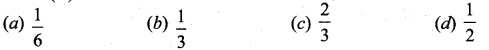Solution:
(b)
Hint: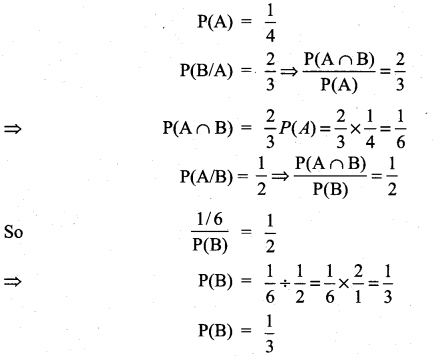Question 22.
In a certain college 4% of the boys and 1% of the girls are taller than 1.8 meter. Further 60% of the students are girls. If a student is selected at random and is taller than 1.8 meters, then the probability that the students is a girls is ………….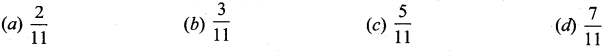Solution:
(b)
Hint: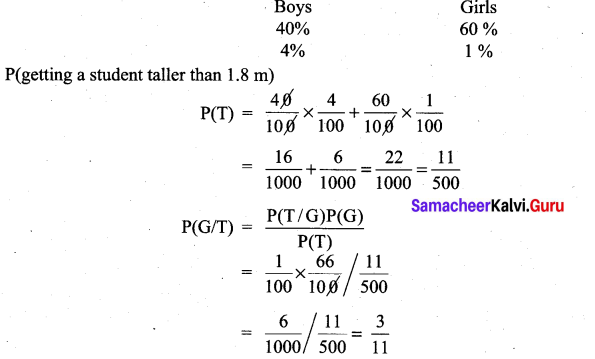Question 23.
Ten coins are tossed. The probability of getting at least 8 heads is …………….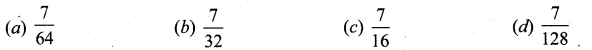Solution:
(d)
Hint:
When 10 coins are tossed, No. of element in sample space
n(S) = 210 = 1024
Probability of getting atleast 8 heads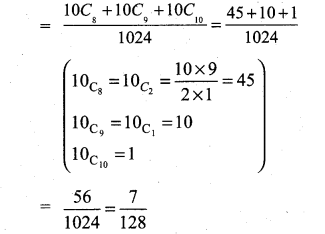Question 24.
The probability of two events A and B are 0.3 and 0.6 respectively. The probability that both A and B occur simultaneously is 0.18. The probability that neither A nor B occurs is …………….
(a) 0.1
(b) 0.72
(c) 0.42
(d) 0.28
Solution:
(d)
Hint:
P(A) = 0.3, P(B) = 0.6
P(A ∩ B) = 0.18
So P(A ∪ B) = P(A) + P(B) – P(A ∩ B)
= 0.3 + 0.6 – 0.18
= 0.9 – 0.18 = 0.72
P(A’ ∩ B’) = P[(A ∪ B)’] = 1 – P(A ∪B)
= 1 – 0.72 = 0.28

Question 25.
If m is a number such that m≤ 5, then the probability that quadratic equation 2x2 + 2mx + m + 1 = 0 has real roots is ………….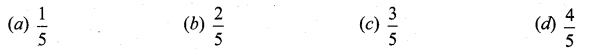Solution:
(c)
Hint:
2x2 + 2mx + m + 1 = 0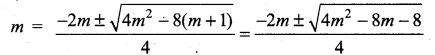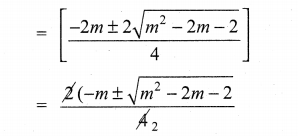roots are real ⇒ m2 – 2m – 2 ≥ 0
Here m ≤ 5 ⇒ n(S) = 5
When m= 1,m2 – 2m – 2
When m = 2, m2 – 2m- 2
When m = 3, m2 – 2m – 2
When m = 4, m2 – 2n- 2
When m = 5, m2 – 2m – 2
⇒ n{A) = 3 and so P(A) = $$\frac{3}{5}$$